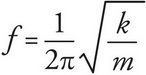# AP Physics 1 Question 254: Answer and Explanation

### Test Information

Question: 254

9. A block with a mass of 4 kg is attached to a spring on the wall that oscillates back and forth with a frequency of 4 Hz and an amplitude of 3 m. What would the frequency be if the block were replaced by one with one-fourth the mass and the amplitude of the block is increased to 9 m ?

• A. 4 Hz
• B. 8 Hz
• C. 12 Hz
• D. 24 Hz

The frequency of a spring-block simple harmonic oscillator is independent of the amplitude. The equation for the frequency of a spring-block simple harmonic oscillator is. The frequency is inversely proportional to the square root of the mass, so decreasing the mass of the block by a factor of 4 would increase the frequency by a factor of 2.